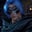Related Tags

java
python
leetcode

# LeetCode: How to rotate an image clockwiseGutha Vamsi Krishna

### Problem

You are given an $n$ x $n$ 2D matrix representing an image, and you need to rotate the image clockwise by 90 degrees. You have to rotate the image in place, i.e., you have to modify the input 2D matrix directly. Do not allocate another 2D matrix and perform the rotation.

### Algorithm

1. Switch the rows up and down. Let’s say we have $n$ rows: switch the $1^{st}$ row and the $n^{th}$ row, the $2^{nd}$ row and the $(n-1)^{th}$ row, and so on.
2. Apply transpose on the resultant matrix.

The transpose of a matrix is obtained by changing its rows into columns and its columns into rows.

The given matrix
1 of 19

### Code

class Solution {
public void rotate(int[][] matrix) {
if(matrix == null || matrix.length == 0 || matrix.length == 0) return;
int rows = matrix.length;
int cols = matrix.length;

for(int first=0,last=rows-1; first<last; first++, last--){
int[] tmp = matrix[first];
matrix[first] = matrix[last];
matrix[last] = tmp;
}

for(int i=0;i<rows;i++){
for(int j=i+1;j<cols;j++){
int tmp = matrix[i][j];
matrix[i][j] = matrix[j][i];
matrix[j][i] = tmp;
}
}

}
}

RELATED TAGS

java
python
leetcode

CONTRIBUTORGutha Vamsi Krishna
RELATED COURSES

View all Courses

Keep Exploring

Learn in-demand tech skills in half the time